the arc of software bends towards understanding

This post is the spiritual predecessor to Flipping Burgers in coBurger King.

What does it mean for something to be dual? A category theorist would say, “It’s the same thing, but with all the arrows flipped around.” This answer seems frustratingly vague, but actually it’s quite precise. The only thing missing is knowing what arrows flip around! If you know the arrows, then you know how to dualize. In this post, I’d like to take a few structures that are well known to Haskellers, describe what the arrows for this structure look like, and then show that when we flip the arrows, we get a dual concept.

### Products and sums

Suppose you have some data of the type Either a b. With all data, there are two fundamental operations we would like to perform on them: we’d like to be able to construct it and destruct it. The constructors of Either are the Left :: a -> Either a b and Right :: b -> Either a b, while a reasonable choice of destructor might be either :: (a -> r) -> (b -> r) -> Either a b -> r (case analysis, where the first argument is the Left case, and the second argument is the Right case). Let’s draw a diagram: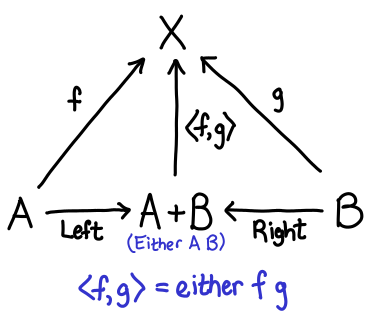I’ve added in two extra arrows: the represent the fact that either f g . Left == f and either f g . Right == g; these equations in some sense characterize the relationship between the constructor and destructor.

OK, so what happens when we flip these arrows around? The title of this section has given it away, but let’s look at it: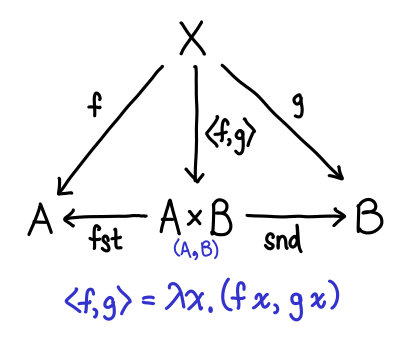Some of these arrows are pretty easy to explain. What used to be our constructors (Left and Right) are now our destructors (fst and snd). But what of f and g and our new constructor? In fact, \x -> (f x, g x) is in some sense a generalized constructor for pairs, since if we set f = const a and g = const b we can easily get a traditional constructor for a pair (where the specification of the pair itself is the arrow—a little surprising, when you first see it):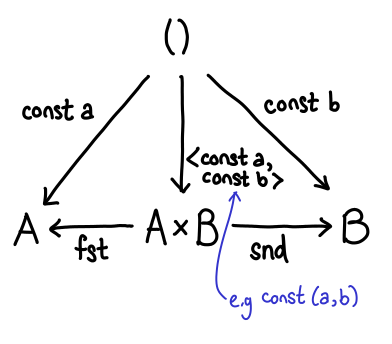So, sums and products are dual to each other. For this reason, sums are often called coproducts.

(Keen readers may have noticed that this presentation is backwards. This is mostly to avoid introducing \x -> (f x, g x), which seemingly comes out of nowhere.)

### Top and bottom

The unit type (referred to as top) and the bottom type (with no inhabitants) exhibit a duality between one another. We can see this as follows: for any Haskell type, I can trivially construct a function which takes a value of that type and produces unit; it’s const ():Furthermore, ignoring laziness, this is the only function which does this trick: it’s unique. Let’s flip these arrows around: does there exist a type A for which for any type B, there exists a function A -> B? At first glance, this would seem impossible. B could be anything, including an uninhabited type, in which case we’d be hard pressed to produce anything of the appropriate value. But wait: if A is uninhabited, then I don’t have to do anything: it’s impossible for the function to be invoked!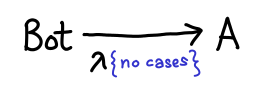Thus, top and bottom are dual to one another. In fact, they correspond to the concepts of a terminal object and an initial object (respectively) in the category Hask.

One important note about terminal objects: is Int a terminal object? It is certainly true that there are functions which have the type forall a. a -> Int (e.g. const 2). However, this function is not unique: there's const 0, const 1, etc. So Int is not terminal. For good reason too: there is an easy to prove theorem that states that all terminal objects are isomorphic to one another (dualized: all initial objects are isomorphic to one another), and Int and () are very obviously not isomorphic!

### Folds and unfolds

One of the most important components of a functional programming language is the recursive data structure (also known as the inductive data structure). There are many ways to operate on this data, but one of the simplest and most well studied is the fold, possibly the simplest form a recursion one can use.

The diagram for a fold is a bit involved, so we’ll derive it from scratch by thinking about the most common fold known to functional programmers, the fold on lists:

```data List a = Cons a (List a) | Nil
foldr :: (a -> r -> r) -> r -> List a -> r
```

The first two arguments “define” the fold, while the third argument simply provides the list to actually fold over. We could try to draw a diagram immediately:But we run into a little bit of trouble: our diagram is a bit boring, mostly because the pair (a -> r -> r, r) doesn’t really have any good interpretation as an arrow. So what are we to do? What we’d really like is a single function which encodes all of the information that our pair originally encoded.

Well, here’s one: g :: Maybe (a, r) -> r. Supposing we originally had the pair (f, z), then define g to be the following:

```g (Just (x, xs)) = f x xs
g Nothing = z
```

Intuitively, we’ve jammed the folding function and the initial value into one function by replacing the input argument with a sum type. To run f, we pass a Just; to get z, we pass a Nothing. Generalizing a bit, any fold function can be specified with a function g :: F a r -> r, where F a is a functor suitable for the data type in question (in the case of lists, type F a r = Maybe (a, r).) We reused Maybe so that we didn’t have to define a new data type, but we can rename Just and Nothing a little more suggestively, as data ListF a r = ConsF a r | NilF. Compared to our original List definition (Cons a (List a) | Nil), it’s identical, but with all the recursive occurrences of List a replaced with r.

With this definition in hand, we can build out our diagram a bit more: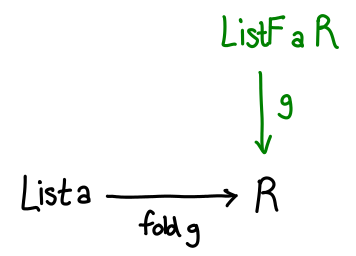The last step is to somehow relate List a and ListF a r. Remember how ListF looks a lot like List, just with r replacing List a. So what if we had ListF a (List a)—literally substituting List a back into the functor. We’d expect this to be related to List a, and indeed there’s a simple, unique function which converts one to the other:

```in :: ListF a (List a) -> List a
in (ConsF x xs) = Cons x xs
in NilF = Nil
```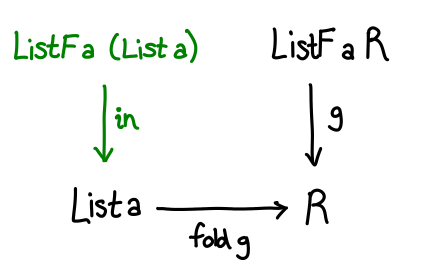There’s one last piece to the puzzle: how do we convert from ListF a (List a) to ListF a r? Well, we already have a function fold g :: List a -> r, so all we need to do is lift it up with fmap.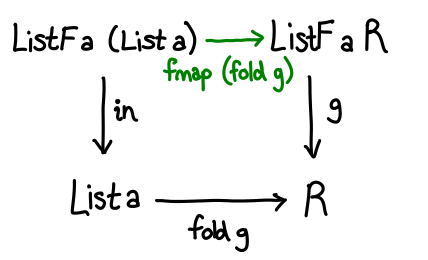We have a commuting diagram, and require that g . fmap (fold g) = fold g . in.

All that’s left now is to generalize. In general, ListF and List are related using little trick called the Mu operator, defined data Mu f = Mu (f (Mu f)). Mu (ListF a) is isomorphic to List a; intuitively, it replaces all instances of r with the data structure you are defining. So in general, the diagram looks like this: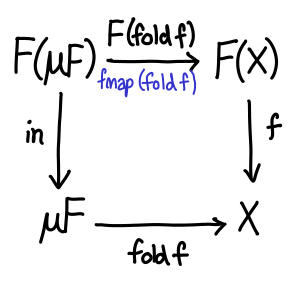Now that all of these preliminaries are out of the way, let’s dualize!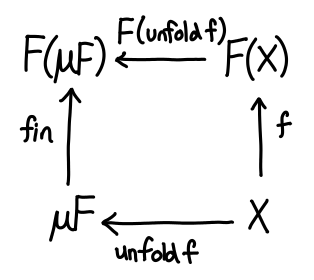If we take a peek at the definition of unfold in Prelude: unfold :: (b -> Maybe (a, b)) -> b -> [a]; the Maybe (a, b) is exactly our ListF!

The story here is quite similar to the story of sums and products: in the recursive world, we were primarily concerned with how to destruct data. In the corecursive world, we are primarily concerned with how to construct data: g :: r -> F r, which now tells us how to go from r into a larger Mu F.

### Conclusion

Dualization is an elegant mathematical concept which shows up everywhere, once you know where to look for it! Furthermore, it is quite nice from the perspective of a category theorist, because when you know two concepts are dual, all the theorems you have on one side flip over to the other side, for free! (This is because all of the fundamental concepts in category theory can be dualized.) If you’re interested in finding out more, I recommend Dan Piponi’s article on data and codata.

### 28 Responses to “Duality for Haskellers”

1.Brett says:

Minor nitpick from a confused beginner, I’m pretty sure your Right type constructor is incorrectly defined. It should be:

Right :: b -> Either a b

Correct?

2.sla says:

I think we miss the essence behind all this categorical stuff.
The duality in category is a must to have property to have well defined category. And showing tha tit there and what are rules of it does not give any explanation or essence of it.
The idea of category being generalized over any set theories flavour, thats what gives rize to duality and all other rules.

So turning arrows and types is just an outcome of this implicit relation.
And your post just stated in many convoluted words thats Duality is there (and must be), but you not really explaining what it is and why it there.

Imo to highlight it, need to show connection between order and set theory. And then Duality becomes natural outcome.

3.Edward Z. Yang says:

Brett: Good catch, it’s been fixed.

sla: Sure, I wouldn’t claim that this post properly describes duality in any sense. But most Haskellers are not intimately familiar with order theory, so I’ve always felt concrete examples in Hask are a bit more helpful for when you actually want to learn about it properly.

4.Ashley Yakeley says:

Do you have a definition in mind for your Hask? For instance, types of finite values with terminating functions?

5.Edward Z. Yang says:

Yes. But whenever someone complains about that, I always point them to this Piponi post: http://blog.sigfpe.com/2009/10/what-category-do-haskell-types-and.html

6.Ashley Yakeley says:

I’m beginning to think there’s a class of platonic Haskell categories, and when one says Hask, one means any given member of this class.

7.Jake McArthur says:

I prefer the presentation of top as (exists x. x) and of bottom as (forall x. x) because explaining them doesn’t require const (which, ignoring some other requirements for top, would work for many other types as long as they are inhabited) or fuzzy arguments about not being inhabited (which feels a bit like cheating since you’re still claiming the function _|_ -> A exists, yet it can’t be defined in Haskell without nontermination in the function body).

8.Edward Z. Yang says:

Jake: Well, part of the reason it works for other types is that there are many types which are isomorphic to unit, no? I guess it’s a reasonable elaboration to explain why Bool is not initial, because yes const works, but the big question is, “Which one?” (It’s not unique…)

As for bottom, yeah, it’s a bit of downside using Haskell. Maybe we should fix that; after all, it works properly in the non-dependent fragments of dependently typed languages.

9.Jake McArthur says:

I think the argument against using undefined to project from initial objects also applies against using const to inject into terminal objects, since the former is actually just the same as (const undefined) anyway.

toTop : a -> (exists x. x)
toUnit : a -> ()

fromBottom : (forall x. x) -> a
fromEmpty : Empty -> a

toTop actually uses its argument, but toUnit simply throws it away. Also, fromBottom uses its argument, but fromEmpty simply throws it away.

10.Edward Z. Yang says:

It is true that the computational content of the functions is different (it is the same in logic: do you prove Bottom -> Bottom axiomatically or with ex falso), but I don’t that makes a difference when viewed categorically?

11.Bo says:

Um, what is X in the first diagram? Is it supposed to say that for any X, we can construct f, g, and such that the diagram commutes?

12.Edward Z. Yang says:

Uh sorry, X is universally quantified over all types (it’s basically ‘r’ in the corresponding type signatures…). Those diagrams may or may not have been drawn way before I wrote the corresponding text. :^)

13.Bo says:

I see, I think I get it now. Basically it says that Z is the sum type of A and B if for all types X and functions (f : A -> X) and (g : B -> X) there exist functions (Left : A -> Z), (Right : B -> Z) and ([f,g] : Z -> X) such that ([f,g] . Left = f) and ([f,g] . Right = g). While the dual definition says that Z is the product type of A and B if for all X, (f : X -> A), (g : X -> B) there exist (fst : Z -> A), (snd : Z -> B) and ([f,g] : X -> Z). Good stuff, thanks. :D

14.DAY says:

Typo:

“in the case of lists, type F a = Maybe (a, r)”, the type variable “r” is missing in the type constructor.

15.DAY says:

Error:

The bottom arrow of the last diagram is bidirectional. It should only be a left arrow.

16.Edward Z. Yang says:

DAY: Thanks, I’ve fixed both.

17.Mike Izbicki says:

One more typo:

g (Just (x, xs) = f x xs

should have two parens

18.Edward Z. Yang says:

Thanks, fixed.

19.Fredrik C says:

Could you point me to a definition of the dual of a *type*? I need it to be able to understand the bottom/top section.

20.Edward Z. Yang says:

Fredrik: There’s no such thing: dualization only ever flips the arrows (functions), not the objects (types). Though maybe there is something that I can explain a little more clearly.

21.Edward Z. Yang says:

Fredrik: I’ve added a clarifying final paragraph, which might help.

22.ben w says:

” a simple, unique function which converts one to the other:”

How unique?

in NilF = Nil
in (ConsF a as) = Cons a \$ Cons a as

also has type ListF a (List a) -> List a, and so do

in NilF = Nil
in (ConsF a as) = case as of Nil -> Nil ; (Cons b bs) -> Cons a \$ Cons b Nil

and uncountably many other functions.

Am I missing something simple? (Given how long it took me to figure out the proper definition of fold from the paragraph introducing ListF—which is not very clear!—quite probably.)

23.Edward Z. Yang says:

Ah yes, I left out one important condition: the diagram must commute! (That is to say, fold g . in == f . fmap (fold f)). I’ve tried to clarify the text a bit.

24.Fredrik C says:

Thanks for the clarification about dual types (namely, there being none). I was led there by the following: “The unit type (referred to as top) and the bottom type (with no inhabitants) are dual to one another.” So what’s meant is one is gotten from the other by flipping the arrow?

The clarification doesn’t quite do it for me, if indeed I have found the correct passage.

25.Fredrik C says:

OK, ignore my last comment. It transpires I had conflated two concepts: “dual” as in “dual statement”, “dual theorem”, and “dual” as in “dual category”, “dual object”.

26.Edward Z. Yang says:

OK, replaced that sentence with more precise wording.

27. […] Haskell, sum types and product types are dual (as are products and coproducts in category theory). You can demonstrate this by implementing f :: […]

28.Rasmus Källqvist says:

Posting this from the far future (compared to the initial date of the post) to say that this has been the most intuitive explanation for F algebras I’ve seen. Really nicely explained!